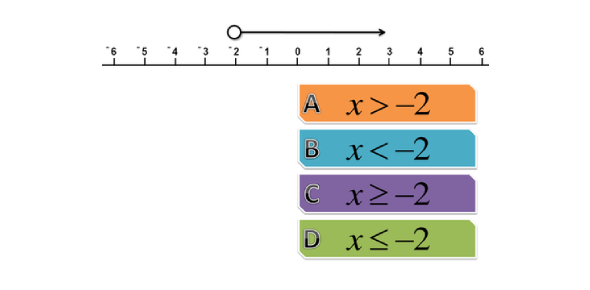# Writing And Graphing Inequalities Quiz!

10 Questions | Total Attempts: 23SettingsDid you study graphic inequalities during your school? Let's check how well do you remember writing and graphing inequalities. All you need is to check the inequality and graphical representation. Take the quiz and review your scores!

• 1.
An open dot is used to graph inequalities solutions with the symbols < or >.
• A.

True

• B.

False

• 2.
A closed dot is used to graph an inequality with what symbols below?  (choose all that apply)
• A.

Option 1

• B.

Option 2

• C.

Option 3

• D.

Option 4

• E.

Option 5

• 3.
If you were to graph X < 10. You would need to draw an open dot at the number 10 and shade the number line to the left.
• A.

True

• B.

False

• 4.
A number less than 6. Choose the correct inequality below that describes the above situation.
• A.

X > 4

• B.

X < 6

• C.

6 < x

• D.

X > 6

• 5.
A number is at least 7. Choose the correct inequality below that describes the above situation.
• A.

X >= 7

• B.

X < 7

• C.

X <= 7

• D.

X > 7

• 6.
Graph the solution to the inequality where a number is greater than or equal to the number 2.
• A.

Option 1

• B.

Option 2

• C.

Option 3

• D.

Option 4

• E.

Option 5

• 7.
Graph the solution to the inequality below. K≤2
• A.

Option 1

• B.

Option 2

• C.

Option 3

• D.

Option 4

• E.

Option 5

• 8.
Graph the solution to the inequality below. k≤3
• A.

Option 1

• B.

Option 2

• C.

Option 3

• D.

Option 4

• E.

Option 5

• 9.
Write an inequality that describes the situation below. A number fewer than 9.
• A.

X >= 9

• B.

X<9

• C.

X <= 9

• D.

X > 9

• 10.
Write an inequality that depicts the situation below. The cost of a shirt exceeds \$22. (let c represent all possible prices of the shirt)
• A.

C < 22

• B.

C <= 22

• C.

C > 22

• D.

C >= 22

Related TopicsBack to top